# Transforms

Provides a demonstration of using Fourier Transforms and Hilbert Transform through JDSP

## Forward Fourier Transform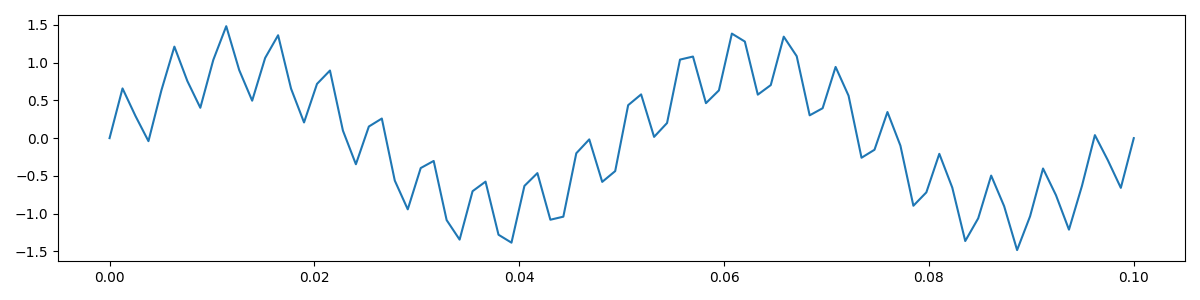##### Signal Used for this Demo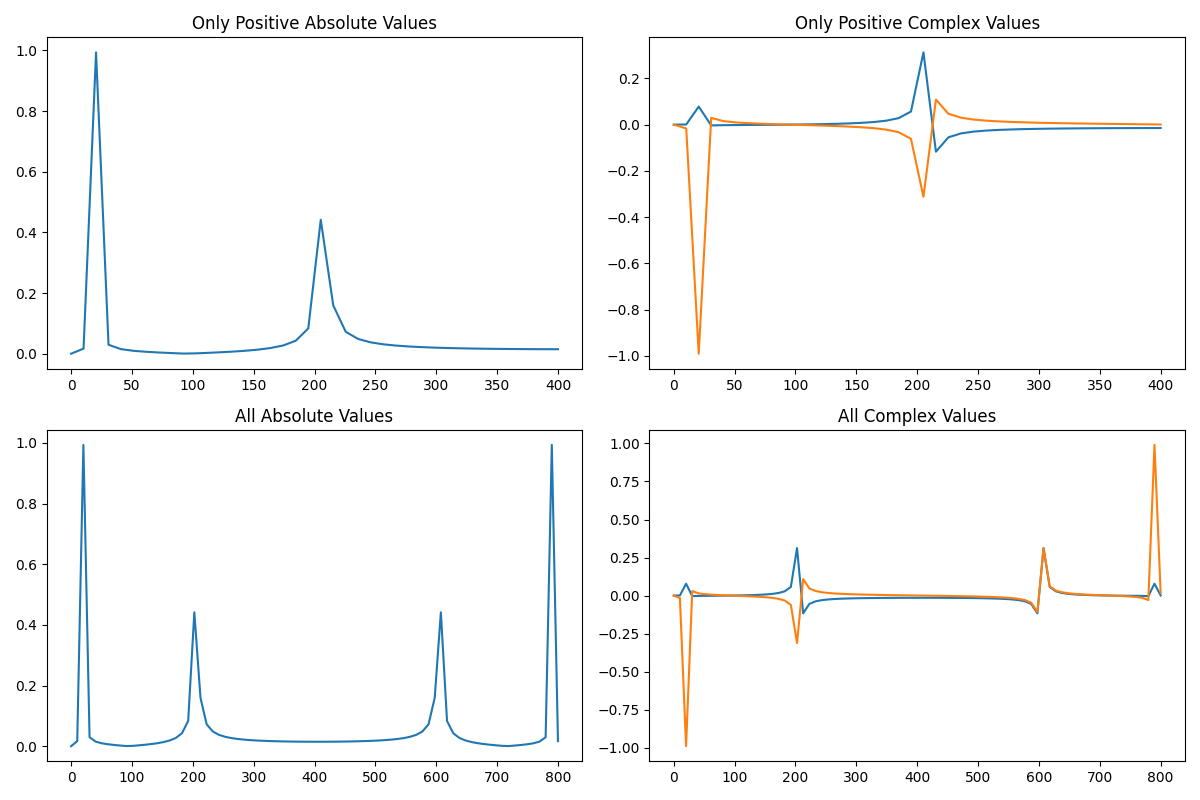#### Only Positive Complex Values

```                    ```
_Fourier ft = new DiscreteFourier(signal);
// OR
_Fourier ft = new FastFourier(signal);
ft.transform();
boolean onlyPositive = true;
double[] out = ft.getMagnitude(onlyPositive); //Positive Absolute
```
```
```                    ```
_Fourier ft = new DiscreteFourier(signal);
// OR
_Fourier ft = new FastFourier(signal);
ft.dft();
boolean onlyPositive = true;
double[][] out = ft.getComplex2D(onlyPositive); //Positive Complex
```
```

#### All Complex Values

```                    ```
_Fourier ft = new DiscreteFourier(signal);
// OR
_Fourier ft = new FastFourier(signal);
ft.dft();
boolean onlyPositive = false;
double[] out = ft.getMagnitude(onlyPositive); //Full Absolute
```
```
```                    ```
_Fourier ft = new DiscreteFourier(signal);
// OR
_Fourier ft = new FastFourier(signal);
ft.dft();
boolean onlyPositive = false;
double[][] out = ft.getComplex2D(onlyPositive); //Full Complex
```
```

## Inverse Fourier Transform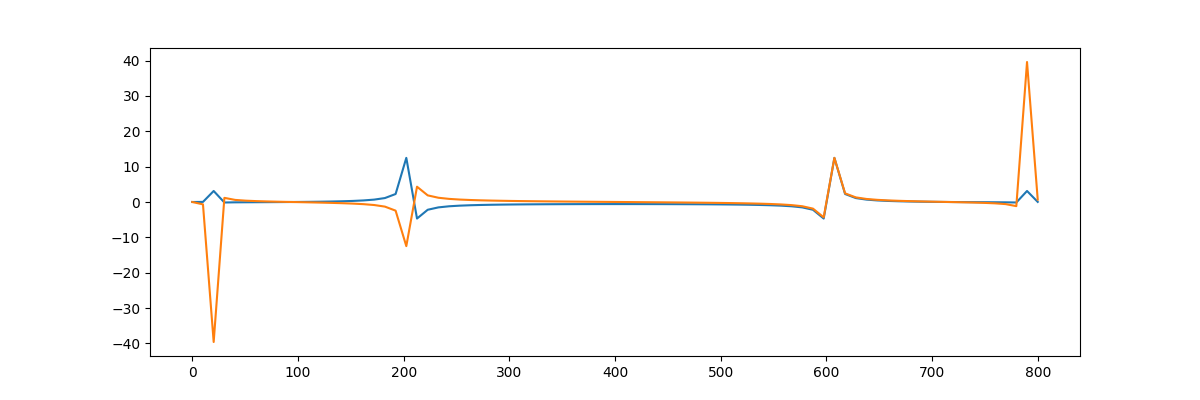##### Sequence Used for this Demo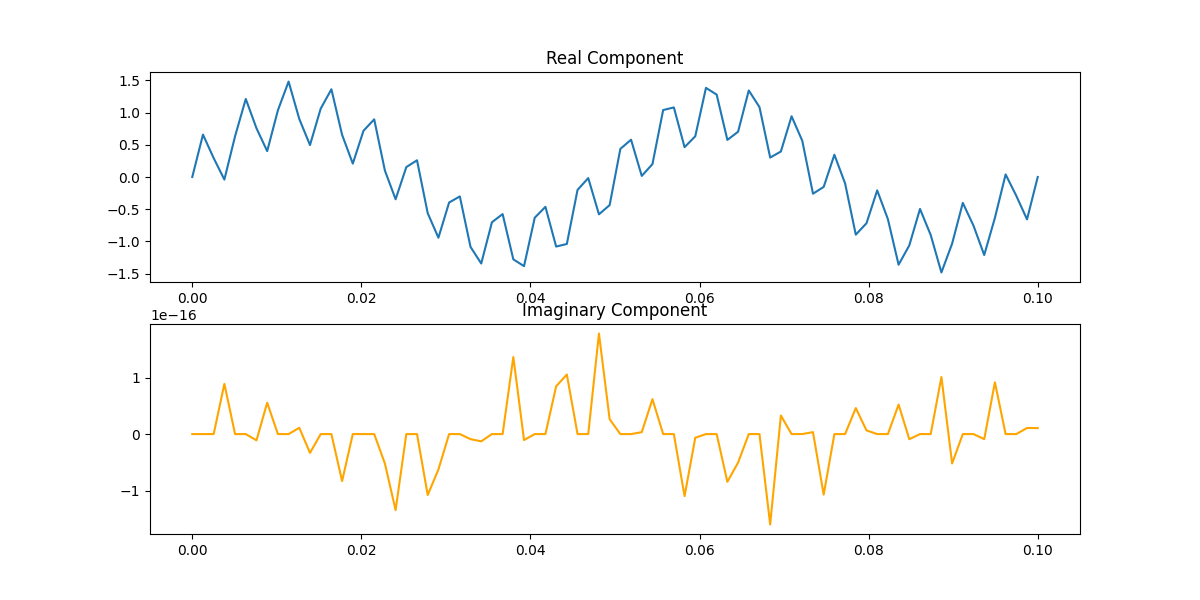```                    ```
_InverseFourier ift = new InverseDiscreteFourier(complexSequence); //If transformed using DFT
// OR
_InverseFourier ift = new InverseFastFourier(complexSequence); //If transformed using FFT
ift.transform();
double[] outReal = ift.getReal(); //To get only the real component
double[] outAbsolute = ift.getMagnitude(); //To get the absolute value of the complex signal
double[][] out = ift.getComplex2D(); // To get the complex signal
```
```

## Forward Short-Time Fourier Transform

```                    ```
int frameLength = 5;
int overlap = 2;
ShortTimeFourier stft = new ShortTimeFourier(signal, frameLength, overlap);
stft.transform();
Complex[][] out = stft.getComplex(false);
_Fourier[] dfts = stft.getOutput();
```
```

## Inverse Short-Time Fourier Transform

```                    ```
int frameLength = 5;
int overlap = 2;
InverseShortTimeFourier istft = new InverseShortTimeFourier(dfts, frameLength, overlap);
istft.transform();
double[] outputReal = istft.getReal();
```
```

## Hilbert Transform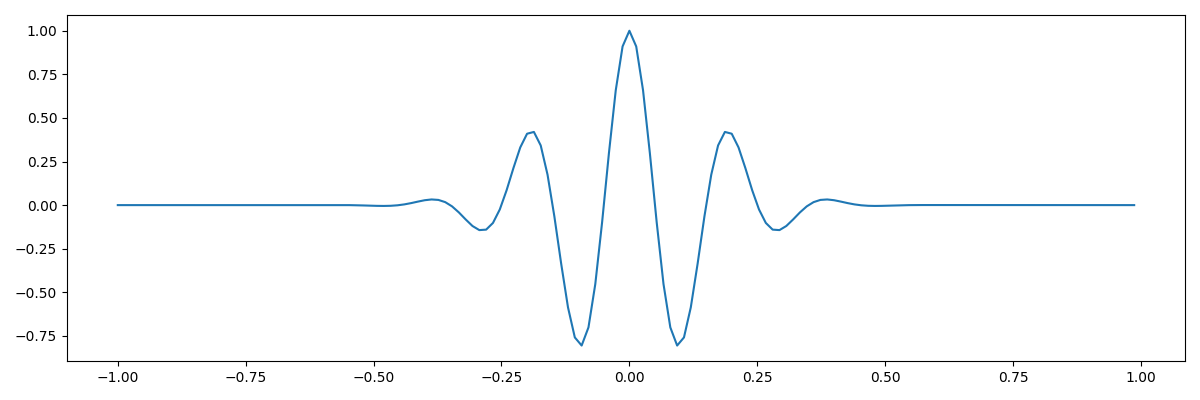##### Sequence Used for this Demo##### Amplitude Envelope of the Signal
```                    ```
Hilbert h = new Hilbert(signal);
h.transform();
// OR
h.transform(true); //To use DFT instead of FFT
double[][] analytical_signal = h.getOutput(); //Returns the analytical signal
double[] envelope = h.getAmplitudeEnvelope();
```
```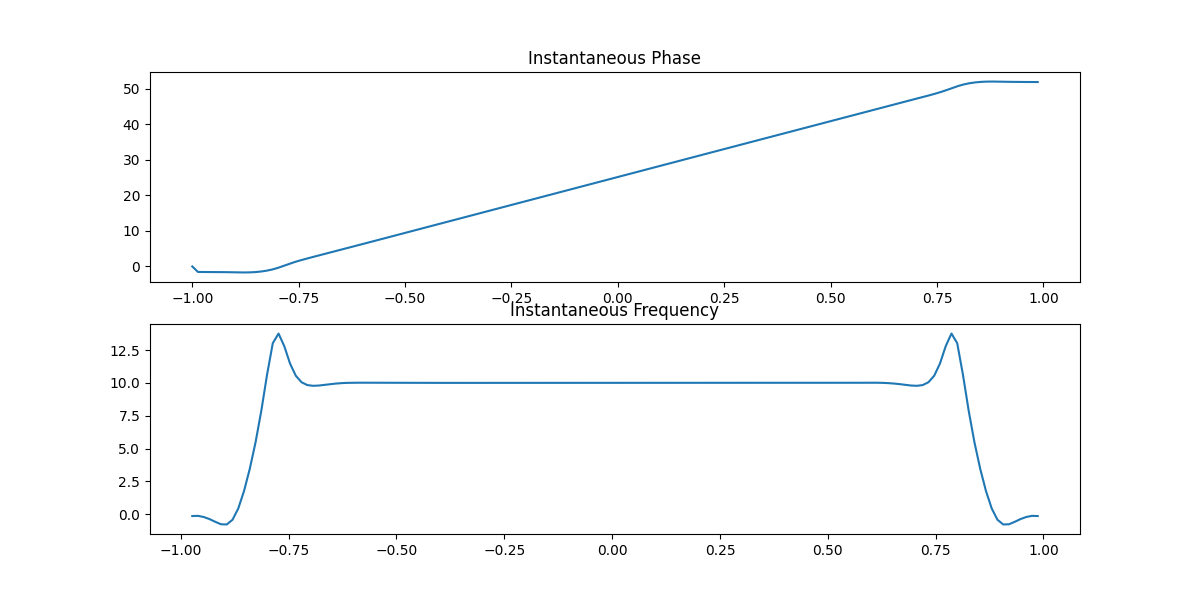##### Instantaneous Phase & Frequency of the Signal
```                    ```
Hilbert h = new Hilbert(signal);
h.transform();
double[] phase = h.getInstantaneousPhase();
int Fs = 150; //Sampling Frequency of the original signal
double[] frequency = h.getInstantaneousFrequency(Fs);
```
```

## Forward Continuous Wavelet Transform

```                    ```
int[] widths = {1,  2,  3,  4,  5,  6,  7,  8,  9, 10};
ContinuousWavelet wR = new ContinuousWavelet(signal, widths);

### For Ricker Wavelet
double ignored_arg = 0.0;
Complex[][] out_cplx = wR.transform(ContinuousWavelet.waveletType.RICKER, ignored_arg);

### For Morlet Wavelet
double morlet_omega0 = 5.0;
Complex[][] out_cplx = wR.transform(ContinuousWavelet.waveletType.MORLET, morlet_omega0);

### For Paul Wavelet
double paul_order = 4.0;
Complex[][] out_cplx = wR.transform(ContinuousWavelet.waveletType.PAUL, paul_order);
```
```

## Inverse Continuous Wavelet Transform

```                    ```
int[] widths = {1,  2,  3,  4,  5,  6,  7,  8,  9, 10};
InverseContinuousWavelet icwt = new InverseContinuousWavelet(out_cplx, widths);

### For Ricker Wavelet
double ignored_arg = 0.0;
double[] signal = icwt.transform(InverseContinuousWavelet.waveletType.RICKER, ignored_arg);

### For Morlet Wavelet
double morlet_omega0 = 5.0;
double[] signal = icwt.transform(InverseContinuousWavelet.waveletType.MORLET, morlet_omega0);

### For Paul Wavelet
double paul_order = 4.0;
double[] signal = icwt.transform(InverseContinuousWavelet.waveletType.PAUL, paul_order);
```
```

## Principal Component Analysis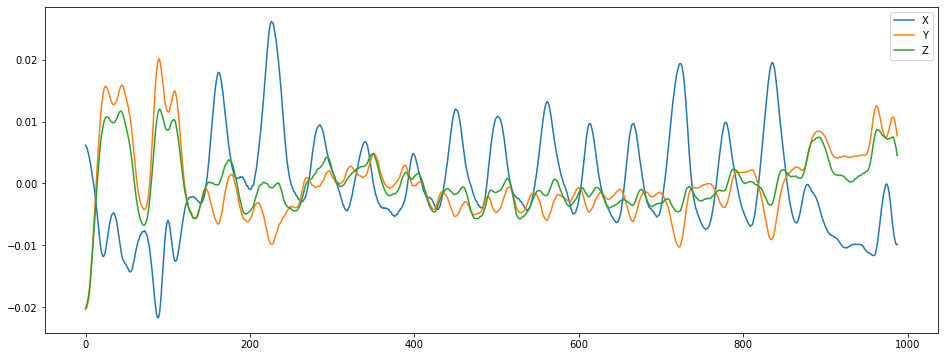##### Signal Used for this Demo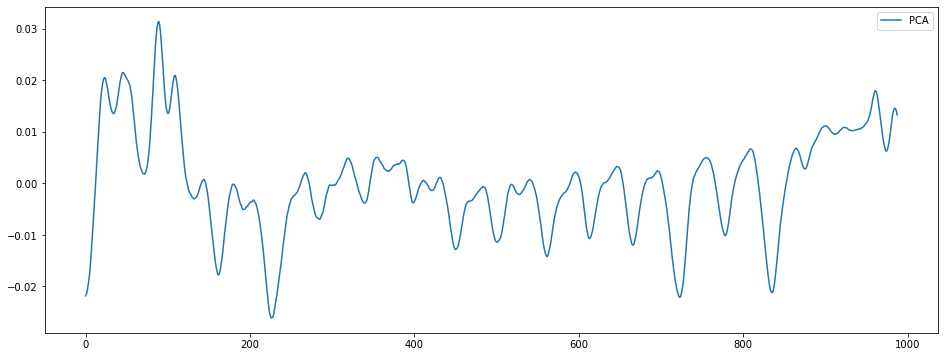##### 1-D Signal after PCA
```                    ```
int dimensions = 1;
PCA p1 = new PCA(signal, dimensions);
p1.fit();
double[][] newSignal = p1.transform(signal); //Returns the reduced signal
```
```